# Convert Inches to Decimal in Microsoft Excel

In this article, we will learn how to convert inches to decimal using Excel as Inches to decimal calculator. An inch is a commonly used term & is the universal unit of measurement. An inch is the name of a unit of length in a number of various systems.

In Excel, we use different conversion to compare results. Here we know the conversion rate from inches to decimal figures.

## Inches to Decimal Calculator

1 inch = (1/12) of a foot = 0.083
6 inch = (6/12) of a foot = 0.5
You must have seen this in size chart of school.

We will be using the above-mentioned conversion here..

Let’s take an example as shown below.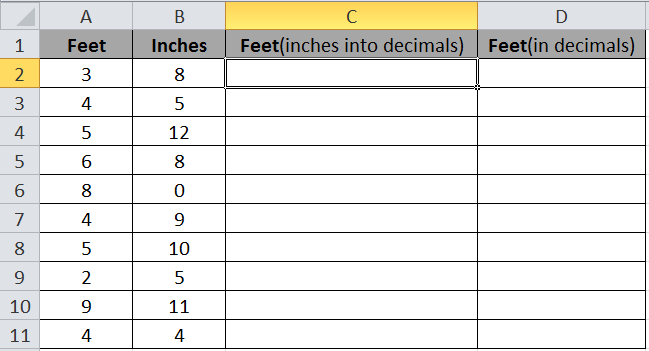Here we have values of feet and inches. We will do it step by step as for everyone’s understanding.

Firstly inches will be converted into decimal and then we will add Feet with Feet(inches into decimal) to get Feet(in decimals).

Use the formula in cell C2.

 =B2/12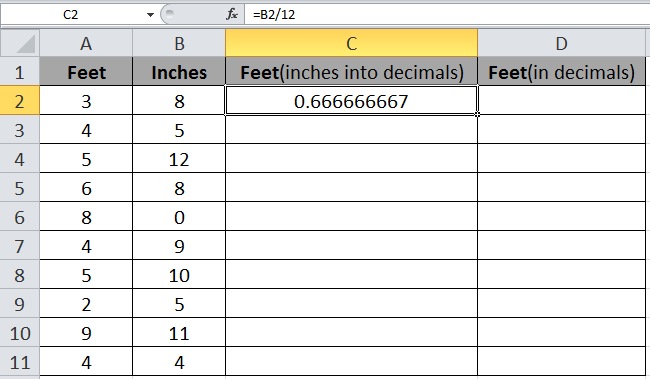Copy the formula in other cells, select the cells taking the first cell where the formula is already applied, use shortcut keys Ctrl + D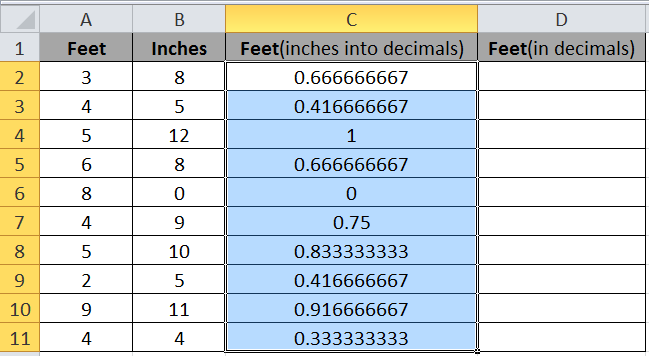Now we just have to add Feet with Feet (inches into decimals) using the formula in cell D2

Formula in D2 cell:

 =C2+A2

We get the below result.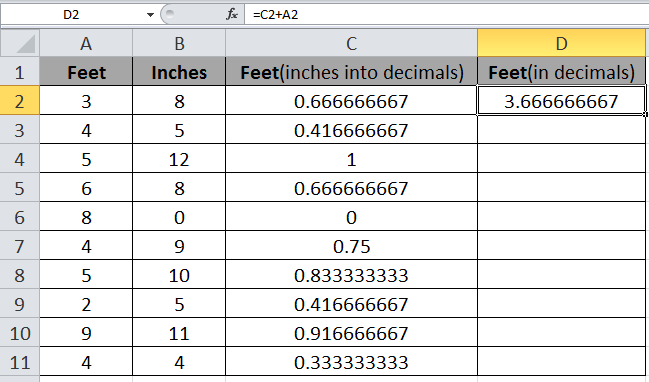Copy the formula in other cells, select the cells taking the first cell where the formula is already applied, use shortcut keys Ctrl + D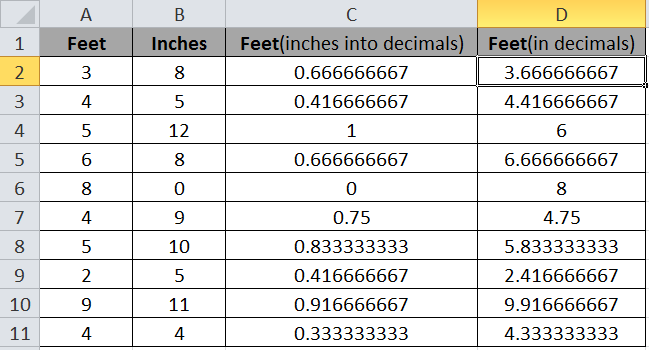As you can see we got our results using the formula.

We can add directly using the formula below.

Formula:

 =A2 + (B2/12)

Q1. How to convert Decimal to Inches?
You can also Feet in decimals to inches in Excel by just multiplying the decimal part with 12, that would be inches.

You can perform these tasks in Excel 2016, 2013 and 2010.

Hope you understood simple addition and division in Excel. Explore more articles on Excel conversion functions here. Please tell us if there’s any doubt in the comment box below. We will definitely help you.

Related Articles:

How to convert Stone to lbs weight in Excel

How to convert minutes to hours and minutes

How to Convert Date and Time from GMT to CST

Popular Articles:

50 Excel Shortcut to Increase Your Productivity

How to use the VLOOKUP Function in Excel

How to use the COUNTIF function in Excel 2016

How to use the SUMIF Function in Excel

1.I like what you have done above.

Can you please try and integrate this formula with the existing formula I have? I'm finding the weight of a value in mm or a weight of a value in feet but I have to change my foot/inch measurements to just feet with decimals. I would rather be able to put 12' 3", for example, instead of googling "how many feet is 147"?"

Current formula below:

=IF(D16>0,(D16/25.4/12)*(E16/25.4/12)*40.84*C16*\$A\$11,IF(F16>0,F16*G16*40.84*\$A\$11*C16,""))

Thanks!

Terms and Conditions of use

The applications/code on this site are distributed as is and without warranties or liability. In no event shall the owner of the copyrights, or the authors of the applications/code be liable for any loss of profit, any problems or any damage resulting from the use or evaluation of the applications/code.